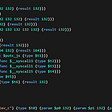# Lambda Calculus: an Elm CLI

## Introduction

The lambda calculus, sometimes described as the world’s smallest programming language, was devised by Alonzo Church in 1932–33 as new foundation for logic. A term (think “phrase”) in the lambda calculus is made up of just three grammatical entities: variables, abstractions, and applications. Variables are things like `x, y, z, ...` . An abstraction (λx.E), where E is a lambda term, defines a function of x. For example, (λx.2x + 1) is the function that doubles whatever x is, then adds 1. The expression (λx.2x + 1) (3) is an application. Its meaning is given by the rule of substitution: in the body of the abstraction, namely the expression 2x + 1, replace every occurrence of x by 3, obtaining 7.

To summarize: we have variables, abstractions, used to define functions, and applications, used to apply functions to data. Application is carried out by the rule of substitution.

In the pure lambda calculus, we have only variables, abstractions, and applications. An expression like 2x + 1 is therefore not part of the pure lambda calculus. It is made up of the integers 1 and 2 and the operations of addition and multiplication. Nonetheless, it is possible to encode the integers in the lambda calculus and with these encodings to define addition, multiplication, and all the rest of arithmetic. Even more is true: anything that can be computed can be expressed in the lambda calculus. This is the Church-Turing thesis. Another way of stating the Church-Turing thesis is that the operation of a universal Turing machine may be encoded in the lambda calculus and, conversely, that one can write a program for a Turing machine that carries out arbitrary computations in the lambda calculus.

## A Lambda Calculator

As a tool to use in my quest to understand the lambda calculus, I wrote a small program in Elm to “run” the lambda calculus. Easier than writing for a Turing machine. Here is a sample session:

`\$ lambdaWelcome! type :help for help> (\x.x) (\y.y)λy.y`

Here the text `\x.x` is the way we write λx.x at the keyboard. We apply this lambda expression to the lambda expression λy.y when we say `(\x.x) (y.y)`. The rule of substitution says “in the body of λx.x, namely x, replace all occurrences of x by λy.y. If we do that, we get λy.y. The lambda term λx.x is the identity function: apply it to anything, and it returns what you give it.

As with any good calculator, we can store things for later use:

`> :let id = \x.xadded id as λx.x> id idλx.x`

Our lambda calculator comes with a “standard library,” as displayed below. The Boolean values of true and false are encoded as λx.λy.x and λx.λy.y, respectively. Encodings of the natural numbers zero, one, two, and three are also given. Working by hand or with the lambda calculator, one finds that `succ zero = one`, `succ one = two,` etc. Thus`succ` is the successor function: the function that adds one to any natural number.

`# Standard Libary# Booleanstrue      \x.\y.xfalse     \x.\y.yand       \p.\q.p q por        \p.\q.p p qnot       \p.p (false) true# Church numeralszero      \s.\z.zone       \s.\z.s ztwo       \s.\z.s s zthree     \s.\z.s s s zisZero    \n.n (\x.zero) truesucc      \n.\f.\x.f(n f x)`

Let’s check out the standard library by doing some Boolean algebra computations

`> and true trueλx.λy.x> and true falseλx.λy.y> and false falseλx.λy.y`

## Implementing the Calculator in Elm

To implement the calculator in Elm, we begin with a definition that captures the notion of a lambda term:

`type Expr    = Var String    | Lambda String Expr    | Apply Expr Expr`

Some examples where we parse a string representation of a lambda term to a value of type `Expr`:

`> :parse xOk (Var "x")> :parse \x.xOk (Lambda "x" (Var "x"))> :parse (\x.x) \y.yOk (Apply (Lambda "x" (Var "x")) (Lambda "y" (Var "y")))`

The relevant code can be found at jxxcarlson/elm-lambda: a package and an app that runs on the command line. There is also the GitHub repo.

Brief summary of the various modules:

Lambda.Expression: the type `Expr` and the function `beta : Expr -> Expr` that does beta reduction, aka computation.

Lambda.Parser: parse strings to Exprs.

Lambda.Defs: the machinery for setting up a dictionary mapping names to string representations of lambda terms so that we can say `and true false` instead of

`(\p.\q.p q p) (\x.\y.x) (\x.\y.y)`

Lambda.Eval: the function `eval: Dict String String -> String -> String` that is used to run the calculator in the `app` folder.

## References

Lambda Calculi, in Internet Encyclopedia of Philosophy

Lambda Calculus (Wikipedia)

Church, Alonzo. 1932. “A Set of Postulates for the Foundation of Logic (Part I).” Annals of Mathematics, 33(2):346–366.

Church, Alonzo. 1933. “A Set of Postulates for the Foundation of Logic (Part II).” Annals of Mathematics, 34(4):839–864.

## Output options

I’ve added some additional output options. The default is `:pretty`. Switch between options using `:named`, `:pretty`, and `:raw:`

`> and true falsefalse> :pretty> and true falseλx.λy.y> :raw> and true false\x.\y.y> :named> and true falsefalse`

Recall that when you type in a lambda expression with no `:command`, the expression is beta-reduced.

## :beta command

The `:beta` command checks for beta-equivalence of two terms:

`> :beta (succ zero) onetrue`

It does alpha-conversion, aka renaming variables:

`> :beta (\x.x) (\a.a)true`

And it has some guards against divergent expressions. Let omega = λx.x x and let Omega = (λx.x x)(λx.x x). Then we have

`> OmegaTOO MANY SUBSTITUTIONS (size2 > 100000). Term may be divergent> :beta Omega OmegaLHS may be divergent`

## More from James Carlson

jxxcarlson on elm slack, http://jxxcarlson.github.io

Love podcasts or audiobooks? Learn on the go with our new app.

## 5 Of The Best Ruby Gems## “E.N.I.M.”: A method to present technical projects in an interview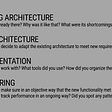## Install Zimbra as Local Project & IntelliJ Remote Debug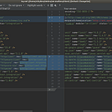## Cloudflare Workers vs AWS Lambda: Detailed Comparison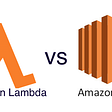## James Carlson

jxxcarlson on elm slack, http://jxxcarlson.github.io

## Building a CLI from scratch with Clapv3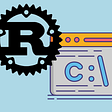## A Beginner’s Guide to Setting up Rust on Windows.## A primer on WebAssembly